×
Log in to StudySoup
Get Full Access to GC&SU - MAT 2100 - Study Guide
Join StudySoup for FREE
Get Full Access to GC&SU - MAT 2100 - Study Guide

Already have an account? Login here
×
Reset your password

GC&SU / Math / Mat 2100 / college statistics study guide

# college statistics study guide Description

##### Uploaded: 11/30/2016
16 Pages 96 Views 1 Unlocks
Reviews

Study Guide Solutions – Baugh – Exam 2 For the discrete probability distribution shown:

x 5 10 20 40 P(X=x ) 25% 12% 15% 48%

## ) What is the sample space?L1  L2  *Create Lists by pressing STAT then Edit #1*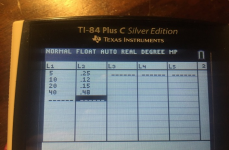(a.) Find the mean (μ)  Sum(L1 * L2) = 24.650 *To calculate Sum,  press 2nd STAT, go to MATH, and Sum is #5. To insert L1, press 2nd 1 and to insert L2, press 2nd 2. *  Or by hand: (5 * .25) + (10 * .12) + (20 * .15) + (40 * .48) = 24.65 Find the variance (σ2)  Sum(L12 * L2) = 846.250 then subtract 24.6502  846.250 – 607.62 = 238.628 Find the standard deviation (σ)  square root the variance  √238.628 = 15.448 (b.) Fill out the table of cumulative probabilities below:

x 5 10 20 40  P(X≤x) 25% 37% 52% 100%

## ) What is the probability of 3 errors in a given hour?*Remember:  Cumulative tables add up to 100%* P(X≤x) means all the percentage values less than or equal to the x value  sooo… x=5  all the percentages that are equal or less than 5  25% x=10  all the percentages that are equal or less than 10  25% + 12% =  37% 1x=20  all the percentages that are equal or less than 20  25% + 12% +  15% = 52% x=40  all the percentages that are equal or less than 40  25% + 12% +  15% + 48% = 100% (c.) Find the expectation value of E[log(X)]  Sum(log(L1) * L2) =  1.259  *Make sure you put L1 in parenthesis or you will get an incorrect  answer* For the Binomial random variable X with n=5, p=0.25 *Remember: A binomial experiment has only 2 possible outcomes, it  consists of “n” repeated trials, the probability of success “p” is the same for  every trial, and the trials are independent meaning that the outcome of one  trial does NOT effect the outcome of another trial* (a.) What is the sample space? *the sample space is the set of  possible outcomes* therefore, if n=5 then the set of possible outcomes have to occur within these 5 trials  { 0, 1, 2, 3, 4, 5 } *Always remember to include 0 in the sample  space when n is numerical* (b.) Find the mean (μ)  n * p  5 * 0.25 = 1.25 Find the variance (σ2)  n * p * q  5 * 0.25 * 0.75 = .938  *q is found by (1 – p), in this case, (1 – 0.25) = 0.75* Fine the standard deviation (σ)  √.938 = .969 (c.) Find P(X = 3)  we want to find the binomialpdf when X=3 only  binomialpdf (n, p, 3) = binomialpdf (5, 0.25, 3) = .088 *IMPORTANT: Use binomialpdf when there is an = sign, use  binomialcdf when there is ≤, ≥, <, or >* *Also, to calculate these press 2nd VARS and its under DISTR. Press the  up arrow to get there faster. It is letter A & B on TI-84* Find P(X < 3)  we want to find the binomialcdf of all probabilities of X  that are less than 3  binomialcdf (5, 0.25, 2) = .869 *Remember:  the x-value is 2 bc we are NOT including 3, but instead only the X  values below it* Find P(X ≥ 10)  we must take into consideration that 10 is outside the  sample space (10 is greater than n=5), so we can automatically  assume the answer is 0. However, if we wanted to make sure… 1 –  binomialcdf (5, 0.25, 10) = 0  2*NOTE: I think he had 1 – binomialcdf (5, 0.25, “9”) written in class;  this is NOT correct bc the “≥” indicates that we are looking for the  binomialcdf of all probabilities greater than or equal to 10, so 10  would be included --- you still get 0 though bc it’s beyond the sample  space* The graph of the probability density function for a continuous random  variable X is shown: *The area of a triangle is ½ base *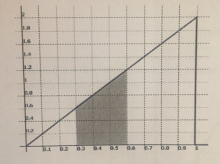height  0.02 per grid square.   (a.) Shade the area corresponding the probability P(0.3 < X < 0.6) &  calculate its value. The area that’s shaded includes all squares that are greater than 0.3  and less than 0.6 while staying inside the density line. If you count the squares, you get 13.5 shaded squares. Then, to find  the probability you multiply 13.5 by 0.02, the area of each grid square.  13.5 * 0.02 = .27, 27% *Note: in class he stated that the probability for any one point is zero* A factory worker is checking the plastic bins to see if the automatic machine  has put the correct part in each bin. There is a 0.02 = 2% chance of a bin  having a wrong part. The worker checks 100 bins each hour and the number  of errors per hour X is a binomial random variable.  Given this scenario we should first try to identify the values of n and p. n = 100 and p = 0.02 (a.) Find the mean (μ)  n * p  100 * 0.02 = 2.0 3Find the variance (σ2)  n * p * q  100 * 0.02 * .98 = 1.96 *q is found by (1 – p), in this case, (1 – 0.02) = 0.98* Find the standard deviation (σ)  √1.96 = 1.40 (b.) What is the probability of 3 errors in a given hour? The  probability of exactly 3 errors in an hour translates into the equation P(X = 3). Since there is an = sign, we use binomialpdf  binomialpdf (n, p, 3) = binomialpdf (100, 0.02, 3) = .1823 What is the probability of at most 3 errors in a given hour? “at most  3 errors” means equal to or less than 3 and translates into the  equation P(X ≤ 3). Since there is a ≤ sign we use binomialcdf and we  include the 3  binomialcdf (n, p, 3) = binomialcdf (100, 0.02, 3) = . 859 *NOTE: his answer is .849 but it is .859* Given Z is a random variable with standard normal distribution ( μ = 0, σ = 1) below is the graph of its probability density function:  *Important: recognize that this is a standard normal distribution, this means  we will be using normalpdf and normalcdf, NOT binomialpdf and binomial  cdf* (a.) Shade in the area corresponding to the probability P(0.5 ≤ Z ≤ 2.0) This means we would shade in all values that are greater than or  equal to 0.5 and those less than or equal to 2.0.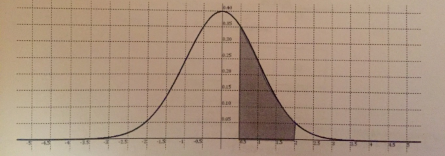(b.) Estimate the number of grid-squares that would fill this area:  11.5  *probably any number between 10.5 and 11.5 would be counted as  correct* 4(c.) Given each grid-square has an area of 0.025, what is the area  estimate? A = 0.025 * 11.5 = .2875 = 28.75%  (d.) Calculate the probability using tables or your calculator: P(0.5 ≤ Z ≤ 2.0) Since there are ≤ signs we use normalcdf  normalcdf (lower,  upper, μ, σ) = normalcdf (0.5, 2.0, 0, 1) = .2858 = 28.58% *Note: μ = 0 and σ = 1 are default values for when the mean and  standard deviation are not directly specified and the graph is a normal  standardized distribution* Given X is a normal random variable with mean μ = 200 and standard  deviation σ = 30. Find the boundary value x such that the probability of X  being greater than x is 20% P(x < X) = 0.2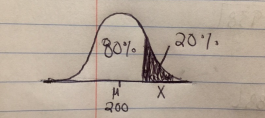*Take into consideration that we are given a probability and are asked to find  an x-value, this should indicate to you that we need to use invNorm* *The invNorm is a way to work backwards from a known probability to find an x-value* Since 20% or 0.2 is shaded, the remaining area is 80% or 0.8  invNorm  (area, μ, σ) = invNorm (.80, 200, 30) = 225.25 *To calculate this, press 2nd VARS and it’s #3 under DISTR* Find z such that the probability that a normal random variable is within z  standard deviations of the mean and is 95%  (Equivalently find that z such that for a standard normal random variable Z,  we have P(-z < Z < z) = 0.95) *Standard normal indicates invNorm will be used* 5If 95% falls between –z and z, then 5% falls beyond the distribution. Half of  this will fall below with –z and half will fall above with +z. So the total area  below +z is 95% + 2.5%. invNorm ( 0.975, 0, 1) = 1.96 *μ = 0 and σ = 1 are default values used when a mean and standard  deviation are not specifically given* 6Statistics – Study Guide Solutions – Baugh Exam 3 1)A single sided fair die has a distribution mean of ux = 3.5 and a distribution standard deviation of σx = 1.707825. You roll the die 50 times and calculate  the sample mean.  n = 50 ux = 3.5 σx = 1.707825 What are the sample mean and sample standard deviation?  ux = 3.5 σx = 1.707825 √50 = 0.24152 What is the probability of P( 3 < X < 4 )? Normalcdf (lower, upper,  mean, sd) Normalcdf (3, 4, 3.5, 0.24152) = 0.96157 2) While rolling a single sided fair die 50 times, you keep track of how many  times you roll either a 1 or 6. Using the fact that the probability for this even  is p = 1/3… n = 50 p = 1/3 Calculate the distribution mean and standard deviation of the sample  proportion.  3(1−13) 1 up = 1/3 σx = √ 50  = 0.06667, 1/15 What is the probability of P( 0.25 < p < 0.40 )?  Normalcdf (lower, upper, mean, sd)  normalcdf (0.25, 0.40, 1/3, 1/15) =  0.7357  73.57% 3) The random variable X has a known standard deviation of σx = 1.5 You  take a sample of 32 trials and calculate a sample mean of X = 7.2 Construct a 90% confidence interval for the mean, ux.  n = 32 σx = 1.5 X = 7.2 ZInterval  Stats, σ = 1.5, X = 7.2, n = 32, C-level = 0.90 = (6.7638,  7.6362)(7.2 - .4362) = 6.7638 & (7.2 - .4362) = 7.6362 4) You carry out a random experiment 100 times and observe a specific  outcome, p = 35% of those times. You are to construct a 90% one sided confidence interval giving an upper bound on the probability of this event  (population proportion).  n = 100 p = 0.35 90%  alpha(α) = 1 - 0.90 = 0.10  ε = δ0.10 √0.35(1−0.35) 100 = 0.061126 0.061126 + 0.35 = 0.411   p < 0.411 5) You wish to test whether a given chip company is cheating its customers.  Their bags claim to contain 13.5oz. Your null hypothesis is the mean bag  contents is the claimed amount. The alternative hypothesis that the mean  value is less.  H0 : ux = 13.5 --------- HA : ux < 13.5 You sample 18 bags and carefully measure the contents. You find the sample  mean is X = 13.25 with a sample standard deviation of Sx = 0.65. Give the  critical region for a 95% significance test. Is the null hypothesis rejected with  your value of 13.25oz?  n = 18 mean = 13.25 standard deviation = 0.65 “If you have to use S you have to use T” 95%  alpha(α) = 1 - 0.95 = 0.05  ε = t0.050.65 18 degrees of freedom (n-1) = 18 – 1 = 17  invT (0.95, 17)  = 1.7396 (also on table) 1.7396 0.65 √18 = 0.2665 Is the null hypothesis rejected with your value of 13.25oz?  13.25 + .2665 = 13.52, greater than 13.25  NO, only reject if X < 13.25.  COMPLETE OUTLINE:Sampling Distributions / Estimation and Confidence Intervals /  Hypothesis Testing  ∙    Sample proportion – the ratio of a class frequency to the total  sample size  ∙    Sample mean – unbiased estimator for the population mean, average. ∙     A one-tailed test will test either if the mean is significantly greater  than x or if the mean is significantly less than x, but not both. A two tailed test does both.  ∙     Unbiased – mean of estimator is the value being estimated; uniformly  minimum variance are unbiased estimators in most cases.  ∙     If S2 is an unbiased estimation of σ2, then S is based on an estimation  of σ.  ∙     Minimal variance – precision  ∙     “If you have to use S then you have to use t, otherwise use σ or z.” ∙     Confidence interval – an interval around the estimator with a  calculated confidence level/error rate ∙     Confidence – is the probability that the procedure yields an internal  containing the value that we seek to estimate.  ∙     Null hypothesis – presumed if no evidence exists, the default.  ∙     Alternative hypothesis – accepted if the null hypothesis is rejected,  influenced by some non-random cause.  ∙    Central Limit Theorem – if you add many independent random  variables, the distance approaches normal distribution.

Ho is not rejected Ho is rejected Ho true Correct Type 1 error “False Positive” Ho false Type 2 error “False Negative” Correct

## What is the probability of at most 3 errors in a given hour?Don't forget about the age old question of dead cell apk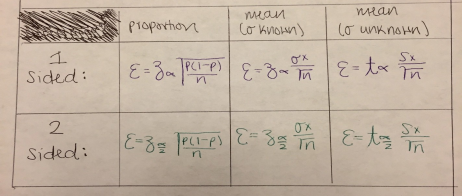Confide nce Level 80% 85% 90% 95% 99% Z-Score 1.28 1.44 1.65 1.96 2.58

We also discuss several other topics like which theory of color vision is best able to explain negative color afterimages
Don't forget about the age old question of according to freud, boys are most likely to experience the oedipus complex during the ________ stage
We also discuss several other topics like - How will you manipulate the independent variable between treatments?
Don't forget about the age old question of calculus 2 study guide
We also discuss several other topics like ecu referencing

Page ExpiredIt looks like your free minutes have expired! Lucky for you we have all the content you need, just sign up here